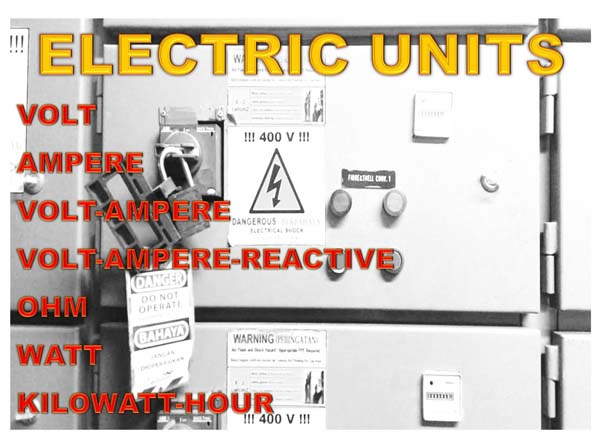# Various Units in electricity, symbols and the correlation

In electricity, we know various units that are used for various types of electrical measurement results, such as electric current, electric power, electric voltage, electrical resistivity, frequency, electricity usage, and others

In this article, we will try to explain various electrical units that are often used to show the results of a measurement in electricity, symbols, correlations and explanations

## Explanation of Various Electric Units and the correlationElectric Units, symbols and the Correlations

VOLTAGE (V)
VOLT is the unit of Voltage (simbolyzed "V"), VOLT is usually symbolized by the letter V (Volt), Electric voltage is an electrical units that is most basic in electricity.

Voltage is a Potential Difference between 2 points generated from an electricity source, Electricity occurs because of proton and electron charges that have different charge or potential difference, Electricity has no voltage if there is no potential difference between the two intermediate points, There are 2 types of voltage, namely:

VDC (Voltage in Direct Current electricity)
Some voltage on DC electricity, including: 1,5 Vdc, 9Vdc, 12Vdc, 24Vdc and others, Examples of sources of electricity that produce DC electricity, namely Battery, In addition, DC power can also be generated by rectifying AC power using an adapter, or From DC Generator

VAC (Voltage ini Alternating Current electricity)
Some voltage on AC Electricity, namely: 110Vac, 220Vac, 380Vac, 415Vac, and others, AC mains voltage is generated from a power source which is commonly called a Generator, AC voltage can also be converted into a DC voltage using a Converter.
"1 KV (KiloVolt) = 1000 Volt"

POWER (P)
Apparent Power (S)
Apparent Power is Measured in Volt Ampere Units, (simbolyzed "VA"), VA is Calculation of Volt (Voltage) and Ampere (Current) from Written specification values
. "1 KVA (Kilo Volt Ampere) = 1000 VA"

Reactive Power (Q)
Reactive Power is Measured in Volt Ampere Reactive Units, (simbolyzed "VAR"), VAR is generated by Electrical Equipment that produce harmonic power.
"1 KVAR (Kilo Vol Ampere Reactive) = 1000 VAR"

Active Power (P)
Active Power is Measured in Watt units, (simbolyzed 'W"), Watt is Calculation of VA and Cosphi (Power Factor).
"1 KW (Kilo Watt) = 1000 Watt"

Power Factor (Cosphi)
Power Factor is the Ratio of Active Power and Apparent Power, (symbolyzed "cos ϕ")
• Cos ϕ = Active Power / Apparent Power
• Active Power = Apparent Power x Cos ϕ

CURRENT (I)
Current is Measured in Ampere Units, (symbolyzed "A"), Electric current is the amount of electric charge caused by the movement of electrons flowing through a conductor in an electric circuit in time units.

Electric current occurs if there is an electrical voltage and resistance or electrical load connected in a circuit, In other words, electric current will not exist if there is no voltage and resistance connected in an electrical circuit, For example, an electric current will flow from the switch to the lamp when we turn on the light switch, then when we switch off, no electric current flows. (The lamp is included in the type of electrical load or resistance)

RESISTANCE (R)
Resistance is Measured in Ohm units (symbolyzed "Ω"), Resistance is anything that generates an electric current when an electric voltage is passed. The size of the electric current generated depends on the size of the electrical voltage and the size of the resistance (Ohm) through which. Ohm's Law and its explanation

V = I x R
• V = Voltage in volts
• I = Current (Intensity in Amperes)
• R = Resistance in Ohms (Ω)

POWER CONSUMPTION
POWER CONSUMPTION is Measured in KiloWatt Hour units (symbloyzed "KWh")

FREQUENCY (F)
Frequency is Measured in Hertz units (symbolyzed "Hz"), Hertz the number of electric waves that occur in one second.

My Electrical Diary